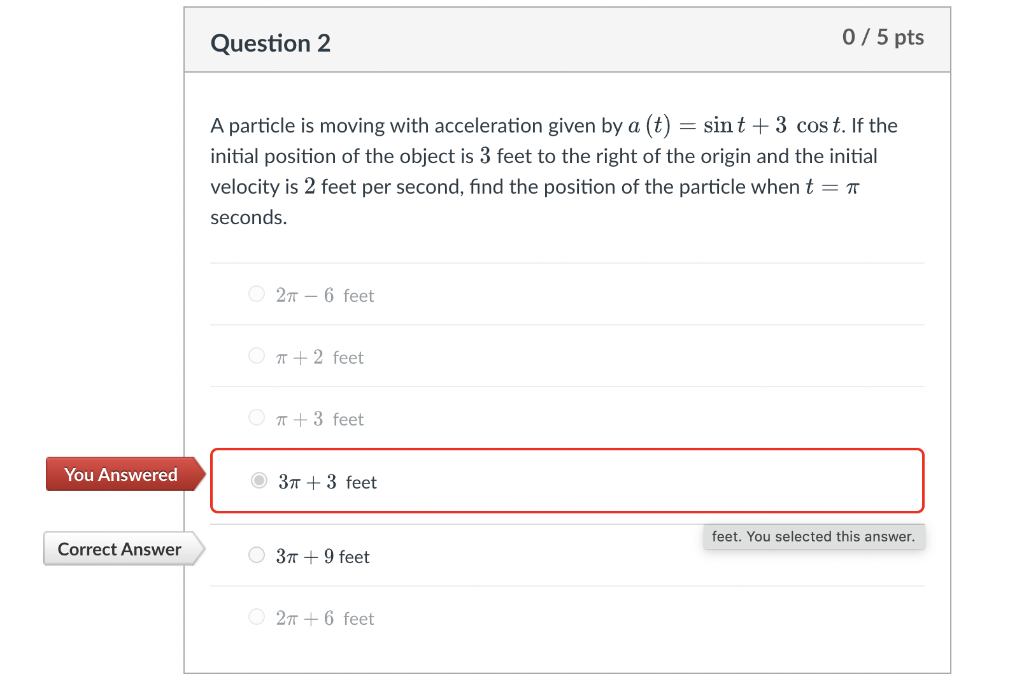Home / Expert Answers / Calculus / a-particle-is-moving-with-acceleration-given-by-a-t-sin-t-3-cos-t-if-the-initial-positio-pa860

# (Solved): A particle is moving with acceleration given by $$a(t)=\sin t+3 \cos t$$. If the initial positio ...A particle is moving with acceleration given by $$a(t)=\sin t+3 \cos t$$. If the initial position of the object is 3 feet to the right of the origin and the initial velocity is 2 feet per second, find the position of the particle when $$t=\pi$$ seconds. $$2 \pi-6$$ feet $$\pi+2$$ feet $$\pi+3$$ feet $$3 \pi+3$$ feet $$3 \pi+9$$ feet $$2 \pi+6$$ feet

We have an Answer from Expert